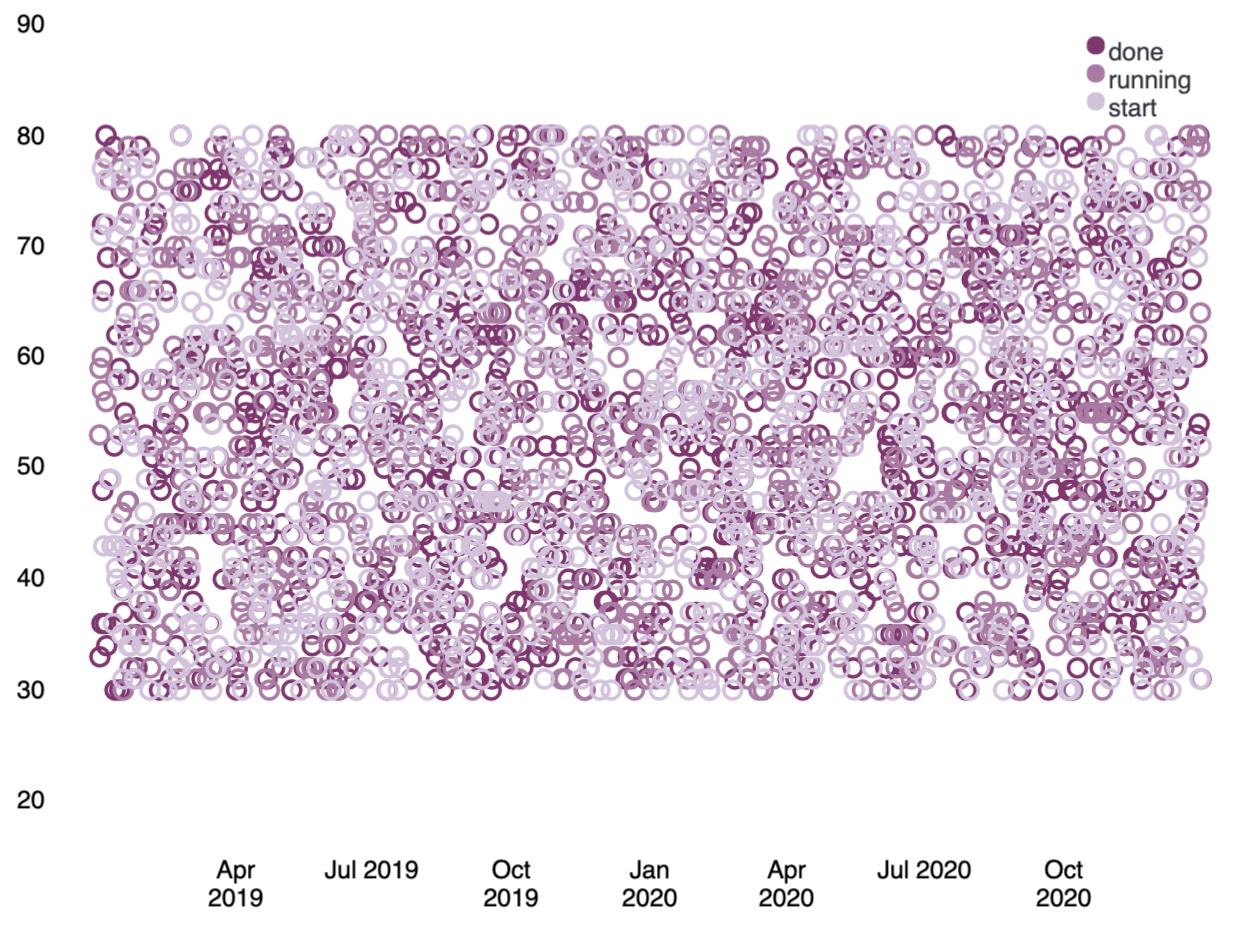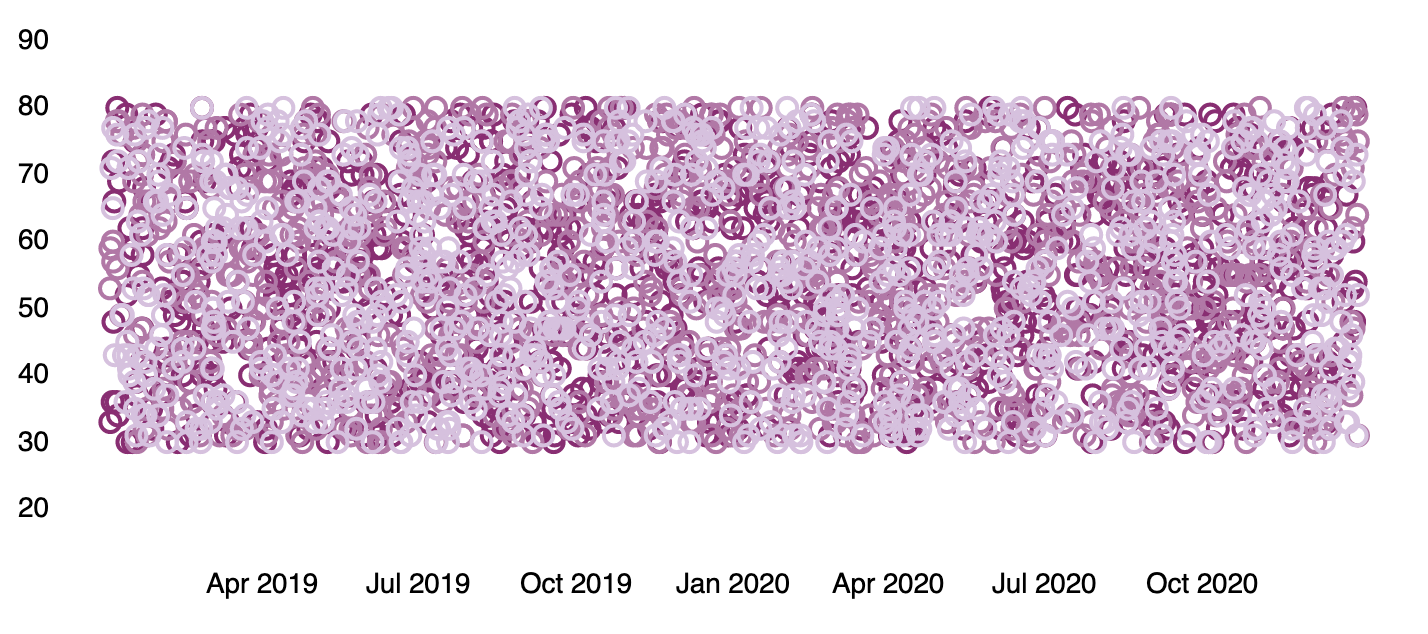You can substitute one function for another to change the output. For example, you could change the visualization by swapping out the pie function for another renderer, a function that returns a `render` data type.

Let’s change that last pie chart into a bubble chart by replacing the pie function with the plot function. This is possible because both functions can accept a `pointseries` data type as their context. Switching the functions will work, but it won’t produce a useful visualization on its own since you don’t have the x-axis and y-axis defined. You will also need to modify the pointseries function to change its output. In this case, you can change the `size` argument to `y`, so the maximum price values are plotted on the y-axis, and add an `x` argument using the `@timestamp` field in the data to plot those values over time. This leaves you with the following expression and produces a bubble chart showing the max price of each state over time:```filters
| demodata
| pointseries color="state" y="max(price)" x="@timestamp"
| plot
| render```

Similar to the pie function, the plot function takes arguments that control the design elements of the visualization. As one example, passing a `legend` argument with a value of `false` to the function will hide the legend on the chart.```filters
| demodata
| pointseries color="state" y="max(price)" x="@timestamp"
| plot legend=false
| render```